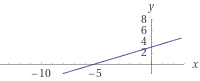# Equal distance

Find the equation for all the points (x, y) that are equal in distance from points A(5,-2) and B(-2,10).

e : 24 y -14 x - 75 = 0

### Step-by-step explanation:Did you find an error or inaccuracy? Feel free to write us. Thank you!

Tips for related online calculators
The line slope calculator is helpful for basic calculations in analytic geometry. The coordinates of two points in the plane calculate slope, normal and parametric line equation(s), slope, directional angle, direction vector, the length of the segment, intersections of the coordinate axes, etc.
Do you have a linear equation or system of equations and looking for its solution? Or do you have a quadratic equation?
Do you want to convert length units?
The Pythagorean theorem is the base for the right triangle calculator.

#### Grade of the word problem:

We encourage you to watch this tutorial video on this math problem: